6351.0.55.001 - Wage Price Index: Concepts, Sources and Methods, 2012
Latest ISSUE Released at 11:30 AM (CANBERRA TIME) 28/11/2012
Page tools:Print AllRSSSearch this Product
Contents >> Chapter 10 - Wage Price Index Calculation in Practice

CHAPTER 10 – WAGE PRICE INDEX CALCULATION IN PRACTICE

INTRODUCTION

10.1 The WPI has been described as a basket of jobs to which employers pay wages and salaries each quarter. As wages and salaries change from one quarter to the next, so too will the total price of the basket.

10.2 The wage price indexes encompass cash payments to employees and include ordinary time earnings, overtime earnings, and bonus, commission and incentive payments, together with the value of any salary sacrificed.

10.3 Price indexes are compiled for the following four wage components:

• ordinary time hourly rates of pay excluding bonuses
• ordinary time hourly rates of pay including bonuses
• total hourly rates of pay excluding bonuses
• total hourly rates of pay including bonuses.

10.4 The wage price indexes can be thought of as being constructed in five major steps:
(i) collecting price data
(ii) deriving a final price for each of the four wage components for each job
(iii) calculating the weighted average prices for each elementary aggregate (EA)
(iv) calculating price relatives and using these to update value aggregates
(v) calculating index numbers.

10.5 Step (i) was outlined in Chapter 8. This chapter provides a description of the other four steps.

DERIVATION OF FINAL PRICES

10.6 The process to derive a final price for each of the four wage components is outlined below. To help explain the process, consider an example job that is paid weekly and has the following attributes:

Ordinary time hourly rate of pay = \$10
Weekly ordinary time standard hours = 38
Weekly overtime standard hours = 2

Ordinary time hourly rate of pay (OTHRP)

10.7 The price of ordinary time hourly rate of pay, excluding bonuses, for each job is simply the base hourly rate. This is referred to as OTHRP and using the example above is \$10.

Ordinary time hourly rate of pay including bonuses (OTHRPIB)

10.8 The hourly bonus price is derived from bonus payment, the frequency of the bonus payment and standard ordinary time hours (unless the frequency of the bonus is the same as the wage and salary payments in which case ordinary time hours for the period are used). For bonuses paid less frequently than quarterly, e.g. annual bonuses, the hourly bonus price is carried forward to subsequent quarters until the next such payment is due.

10.9 Our example job is paid a weekly bonus of \$152 and this is converted to a hourly bonus price of \$4 since the job has weekly ordinary hours of 38.

10.10 To calculate a price inclusive of bonuses, the derived hourly bonus price is added to the ordinary time hourly rate of pay:

(10.1)
OTHRPIB = OTHRP + B

where:
B is the hourly price of bonuses.

10.11 Substituting for our example job:
OTHRPIB = \$10 + \$4
= \$14.

Total hourly rate of pay (THRP)

10.12 The difference between the price for ordinary time hourly rate of pay and the price for total hourly rate of pay is overtime. The pattern of overtime can fluctuate, and this variation is controlled by holding overtime standard hours and the overtime provision constant. Calculation of an overtime provision factor is explained in the following paragraph.

10.13 In addition to deriving the standard overtime hours for the job, an overtime provision factor is derived from data collected in the first quarter. This factor is used to derive an average penalty rate for overtime hours worked and it represents the relationship between the hourly rates for overtime worked and ordinary time. For jobs where the occupant is not eligible to work overtime or where overtime is not being priced (i.e. overtime was not worked in the first quarter the job was included in the survey), the standard overtime hours and overtime provision factor are both set to zero. An example of the calculation used to derive the overtime provision factor is shown below:
1 hour @ \$15 (time and a half) = \$15
1 hour @ \$20 (double time) = \$20
Total overtime paid = \$15 + \$20 = \$35
Total overtime hours = 1 + 1 = 2
Overtime hourly price = \$35 / 2 = \$17.50
Ordinary time hourly rate of pay = \$10
Overtime provision factor = \$17.50 / \$10.00 = 1.75

10.14 The total hourly rate of pay is calculated by combining the overtime hourly rate with the ordinary time hourly rate. To do this, the standard weekly ordinary time and overtime hours are used to weight the wages and salary components.

(10.2)

Ordinary time weight = StdHrs / (StdHrs + VStdHrs )

(10.3)

Overtime weight = VStdHrs / (StdHrs + VStdHrs)

where:
StdHrs is the weekly standard ordinary time hours
VStdHrs is the weekly standard overtime hours.

10.15 Substituting for our example job at paragraph 10.13 which has a weekly overtime standard hours of 2:

Ordinary time weight = 38 / (38 + 2) = 0.95
Overtime weight = 2 / (38 + 2) = 0.05

10.16 The total hourly rate of pay excluding bonuses (THRP) is calculated as:

(10.4)

THRP = (OTHRP x Ordinary time weight) + (Overtime x Overtime weight)

where:
Overtime is the overtime hourly price as described in paragraph 10.13 above.

10.17 Consider the example job at paragraph 10.13. Substituting into the equation for THRP:

THRP = (\$10 x 0.95) + (\$17.50 x 0.05) = \$10.38

Total hourly rate of pay including bonuses (THRPIB)

10.18 The calculation for total hourly rate of pay including bonuses adds in the hourly bonus price to the equation as follows:

(10.5)

THRPIB = (OTHRPIB x Ordinary time weight) + (Overtime x Overtime weight)

10.19 Again using our example job at paragraph 10.13 we have:

THRPIB = ((\$10 + \$4) x 0.95) + (\$17.50 x 0.05) = \$14.18

10.20 To summarise using our example job, the final price for each of the four wage components are:

OTHRP = \$10.00

OTHRPIB = OTHRP + B

= \$14.00

THRP = (OTHRP x Ordinary time weight) + (Overtime x Overtime weight)

= \$10.38

THRPIB = (OTHRPIB x Ordinary time weight) + (Overtime x Overtime weight)

= \$14.18

WEIGHTED AVERAGE PRICE

10.21 The price for a job is combined with the prices for other jobs to determine the price for each elementary aggregate (EA). EAs are the basic building block of the WPI (see paragraph 7.3). They represent a group of jobs with the same state, sector and industry characteristics. An EA price is calculated by taking a weighted average of prices for the jobs in the EA. These are referred to as the weighted average prices. The weights used take account of both the sampling weight (the population represented by the job) and weekly standard hours for each job. As each index is based on combinations of prices (i.e. ordinary time, overtime and bonus) and standard hours, the calculation of various weighted average prices differs for each index. The calculations used for each weighted average price are outlined below.

Calculation of Weighted Average Price for Ordinary Time Hourly Rate of Pay

10.22 An EA for ordinary time hourly rate of pay is calculated by taking a weighted average of prices for the jobs in the EA. It is calculated using the following method:

(10.6)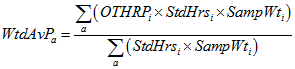where:
WtdAvPa is the weighted average price for EA a
OTHRPi is the ordinary time hourly rate of pay price for job i
StdHrsi is the weekly standard ordinary time hours for job i
Sampwti is the sample weight for job i.

10.23 Consider an example EA, which is made up of the following jobs.

TABLE 10.1: CALCULATION OF WEIGHTED AVERAGE PRICEOTHRP StdHrs Sampwt Job 1 \$18 40 200 Job 2 \$15 20 300 Job 3 \$25 38 150

10.24 Substituting the values into the above equation: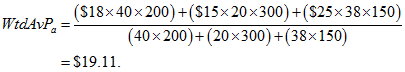Calculation of Weighted Average Price for Total Hourly Rates of Pay

10.25 The EA price is calculated by taking a weighted average of all the job prices in the EA. For total hourly rates of pay, the weight relating to standard hours includes both the ordinary time and overtime standard hours.

(10.7)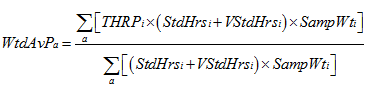where:
WtdAvPa is the weighted average price for EA a
THRPi is the total hourly rate of pay excluding bonuses price for job i.

Calculation of Weighted Average Price for Ordinary Time Hourly Rate of Pay Including Bonuses

10.26 The weighted average price for the index including bonuses is calculated using the same methodology as for the index without bonuses:

(10.8)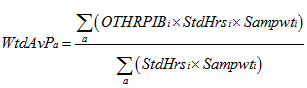where:
WtdAvPa is the weighted average price for EA a
OTHRIBi is the ordinary time hourly rate of pay including bonuses for job i
StdHrsi is the weekly standard ordinary time hours for job i
Sampwti is the sample weight for job i.

Calculation of Weighted Average Price for Total Hourly Rate of Pay Including Bonuses

10.27 Again the weighted average price for the index including bonuses is calculated using the same methodology as for the index without bonuses:

(10.9)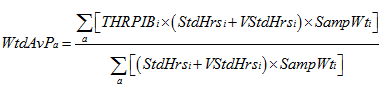where:
WtdAvPa is the weighted average price for EA a
THRPIBi is the total hourly rate of pay including bonuses for job i.

CALCULATION OF PRICE MOVEMENTS FOR ELEMENTARY AGGREGATES

10.28 When calculating the WPI, the Laspeyres methodology is applied. Specifically, prices are compared between the current period and the reference period. In practice, to ensure the most up to date sample (basket) of jobs is used in the calculation of the index the price movement is actually calculated between the current period and the previous period. A price relative (Pt / Pt-1) is calculated for each segment of the labour market (elementary aggregate) which compares the weighted average EA price in the current period with the weighted average EA price in the previous period. Specifically, the price relative (Pt / Pt-1) is equivalent to WtdAvPt / WtdAvPt-1 .

10.29 The estimates of price movements are used to revalue the value aggregates and to value current period prices by applying the period to period price movement to the previous periods value aggregate for each EA using the following formula:

VAt = VAt-1 * (Pt / Pt-1)

(10.10)

The updated value aggregate provides an estimate of the cost of acquiring the reference base quantity of the EA's jobs in the current period. Value aggregates are calculated at the EA level for each of the wage price indexes.

10.30 Table 10.2 below demonstrates the calculation of value aggregates for total hourly rates of pay for three elementary aggregates.

TABLE 10.2: CALCULATION OF VALUE AGGREGATESWEIGHTED AVERAGE PRICES PRICE RELATIVESElementary Aggregate Expenditure Weight P0 P1 P2 P0/P0 P1/P0 P2//P1 1 36 000 13.65 14.36 15.12 1.0000 1.0520 1.0529 2 25 000 12.89 15.54 15.63 1.0000 1.2056 1.0058 3 21 000 11.45 11.62 12.98 1.0000 1.0148 1.1170VALUE AGGREGATES Elementary AggregateVA0 VA1 VA2 136 000 37 873 39 877 225 000 30 140 30 314 321 000 21 312 23 806INDEX CONSTRUCTION

How the indexes are calculated

10.31 In the WPI, index numbers are created by comparing the current period value aggregate with the previous period value aggregate. Whilst different value aggregates are used as inputs for each of the four WPI indexes, the process is essentially the same. This is represented by the following formula:

(10.11)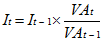where the summation is across all EAs,
It is the index value for the current period
It-1 is the index value for the base period
VAt is the value aggregate in the current period
VAt-1 is the value aggregate in the previous period.

10.32 Each index (Australia, states/territories, sector etc.) represents the amalgamation of a number of EA value aggregates. All of the various wage price indexes are calculated in this manner. Obviously, the combination of EAs and their value aggregates varies depending on the index being compiled.

10.33 The table below demonstrates the index calculation process. If we assume the NSW private sector is split into 3 EAs (1, 2 and 3), Table 10.3 outlines the calculation of the total hourly rates of pay index for the NSW private sector.

 TABLE 10.3: AGGREGATION OF ELEMENTARY AGGREGATES TO FORM AN INDEXVALUE AGGREGATES INDEX Elementary AggregateVA0 VA1 VA2 I0 I1 I2 136 000 37 873 39 877 - - - 225 000 30 140 30 314 - - - 321 000 21 312 23 806 - - - Index100.0 108.9 114.6